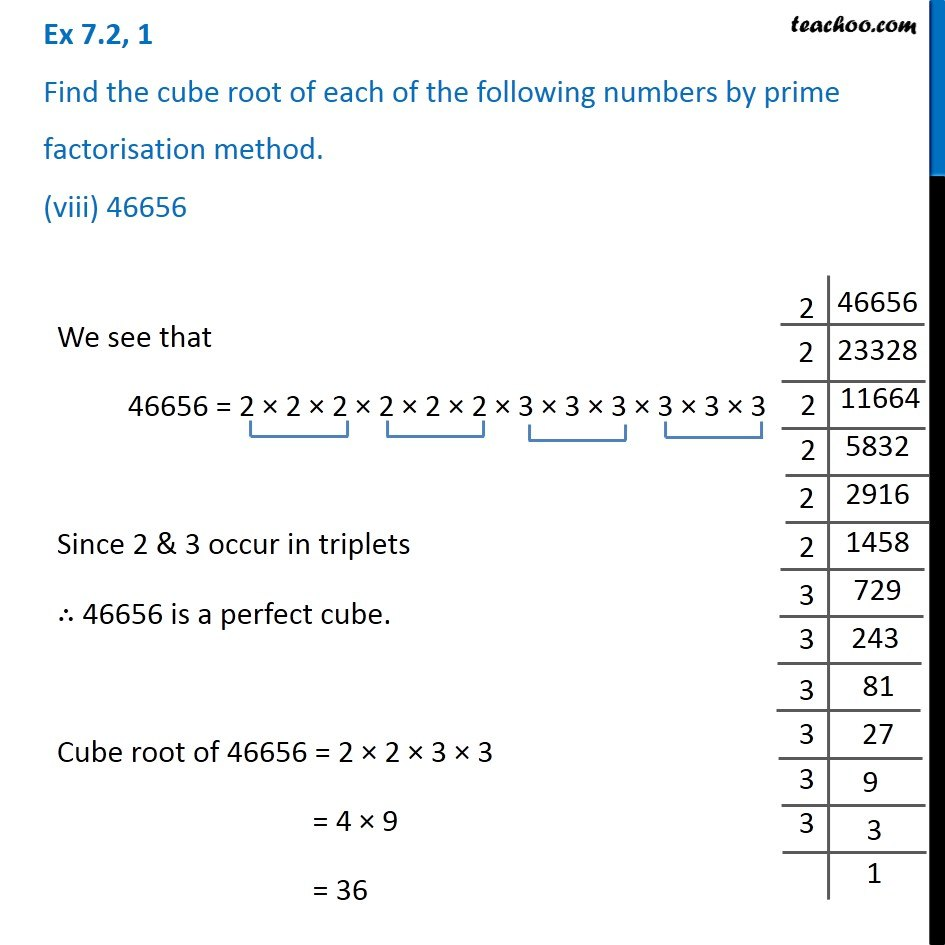Ex 7.2

Chapter 7 Class 8 Cubes and Cube Roots
Serial order wiseGet live Maths 1-on-1 Classs - Class 6 to 12

### Transcript

Ex 7.2, 1 Find the cube root of each of the following numbers by prime factorisation method. (viii) 46656We see that 46656 = 2 × 2 × 2 × 2 × 2 × 2 × 3 × 3 × 3 × 3 × 3 × 3 Since 2 & 3 occur in triplets ∴ 46656 is a perfect cube. Cube root of 46656 = 2 × 2 × 3 × 3 = 4 × 9 = 36